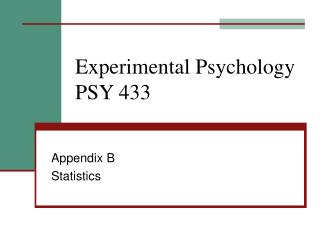DownloadDownload PresentationExperimental Psychology PSY 433

# Experimental Psychology PSY 433

Télécharger la présentation## Experimental Psychology PSY 433

- - - - - - - - - - - - - - - - - - - - - - - - - - - E N D - - - - - - - - - - - - - - - - - - - - - - - - - - -
##### Presentation Transcript

1. Experimental PsychologyPSY 433 Appendix B Statistics

2. Goals for the Experimenter • Create the strongest effect possible: • Increase the number of subjects • Improve the stimuli and task (e.g., increase trials, change manipulation) • Reduce “noise” – unwanted variance: • Control as much as possible • Make sure all subjects have the same experience (except for the manipulation) • Eliminate confounds – the only explanation should be the “alternative explanation

3. Descriptive & Inferential Statistics • Descriptive statistics -- organize, summarize & describe data. • Inferential statistics -- make inferences about a large group based on data from a small portion of those people • The large group is called the population. • The small portion is called the sample. • We generalize from the sample to the population.

4. Samples and Populations • Example: Randomly assign 100 students to 2 groups of 50 • One group gets a drug and the other a placebo. • Test both groups’ memory for 80 words. • Mean # of words recalled: drug = 48, placebo = 42. • What are the samples and what are the populations? • What can be inferred about the population?

5. Null & Alternative Hypotheses • Does the drug improve memory in students? • There are two possibilities: • The drug has no effect and the difference between sample means reflects random chance (null hypothesis) • The drug improves memory and the difference between sample means reflects the presence of two different populations (alternative hypothesis).

6. Hypothesis Testing • We first assume there is no effect of the drug on memory (null hypothesis) • We then look at the difference between sample means & ask: how likely is this difference if the null hypothesis is true? • Small differences are likely (due to chance), so the null hypothesis (no difference) could be true. • Large differences are unlikely, so we reject the null hypothesis and decide the drug most likely did affect memory.

7. Significance Level • We reject the null hypothesis if there is a “large difference” between sample means • But what’s a “large difference?” • A “large difference” is one that would occur less than 5% of the time by chance alone (significance level, or p < .05) • This is called a significant difference.

8. Kinds of Descriptive Statistics • Measures of central tendency – use a single number to describe the group. • Useful for comparing between multiple groups. • Mean, median, mode. • Measures of dispersion – quantifies how much the values are spread out or distant from the mean. • Range • Variance • Standard deviation

9. Inferential Statistics • Used to test difference between means or between a mean and some other number. • Answers the question: could this result have occurred due to chance (normal variability)? • Compares the observed values against what typically occurs with repeated sampling – the normal distribution. • Standard error of the mean – a standard deviation for the means of all possible samples from a population.

10. Kinds of Inferential Tests • Tests for a single group against a known value: • Single group z-test or t-test • Tests for differences between two groups: • Independent groups t-test • Repeated measures (paired groups) t-test • Tests for difference between several groups: • ANOVA – for one IV (one-way) • Repeated measures ANOVA • Multi-factor ANOVA

11. Nonparametric Tests • When data is not normally distributed then the assumptions about what might occur due to chance are different. • Two choices: • Convert data to normal distribution. • Minimize the odd distribution and make it more closely normal by using the rank orders of the observations instead of their actual values. • Slightly different tests are required -- each test has a non-parametric equivalent.